﻿

### Heegaard splittings of the Brieskorn homology spheres that are equivalent after one stabilization

#### Abstract

We construct a class of 3-manifolds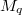$M_q$ which are homeomorphic to  the Brieskorn homology spheres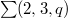$\sum(2,3,q)$, where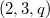$(2,3,q)$ are  relatively prime. Also, we show that$M_q$ is a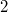$2$-fold cyclic  branched covering of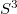$S^3$ over a knot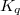$K_q$ which is inequivalent  with torus knot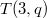$T(3,q)$ for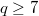$q \ge 7$. Moreover, we show that two  inequivalent Heegaard splittings of$\sum(2,3,q)$ of genus 2  associated with$T(3,q)$ and$K_q$ are equivalent after single  stabilization.

DOI Code: 10.1285/i15900932v20n1p53

Keywords: Crystallization; Heegaard splitting; Crystallization move; Brieskorn homology sphere

Classification: 57M12; 57M15; 57M50

Full Text: PDF